CBSE Class 10 Sample Paper for 2023 Boards - Maths Standard

Class 10
Solutions of Sample Papers for Class 10 Boards

## In a flight of 600km, an aircraft was slowed down due to bad weather. Its average speed for the trip was reduced by 200 km/hr from its usual speed and the time of the flight increased by 30 min. Find the scheduled duration of the flight.

This question is Similar to Ex 4.3 - Chapter 4 Class 10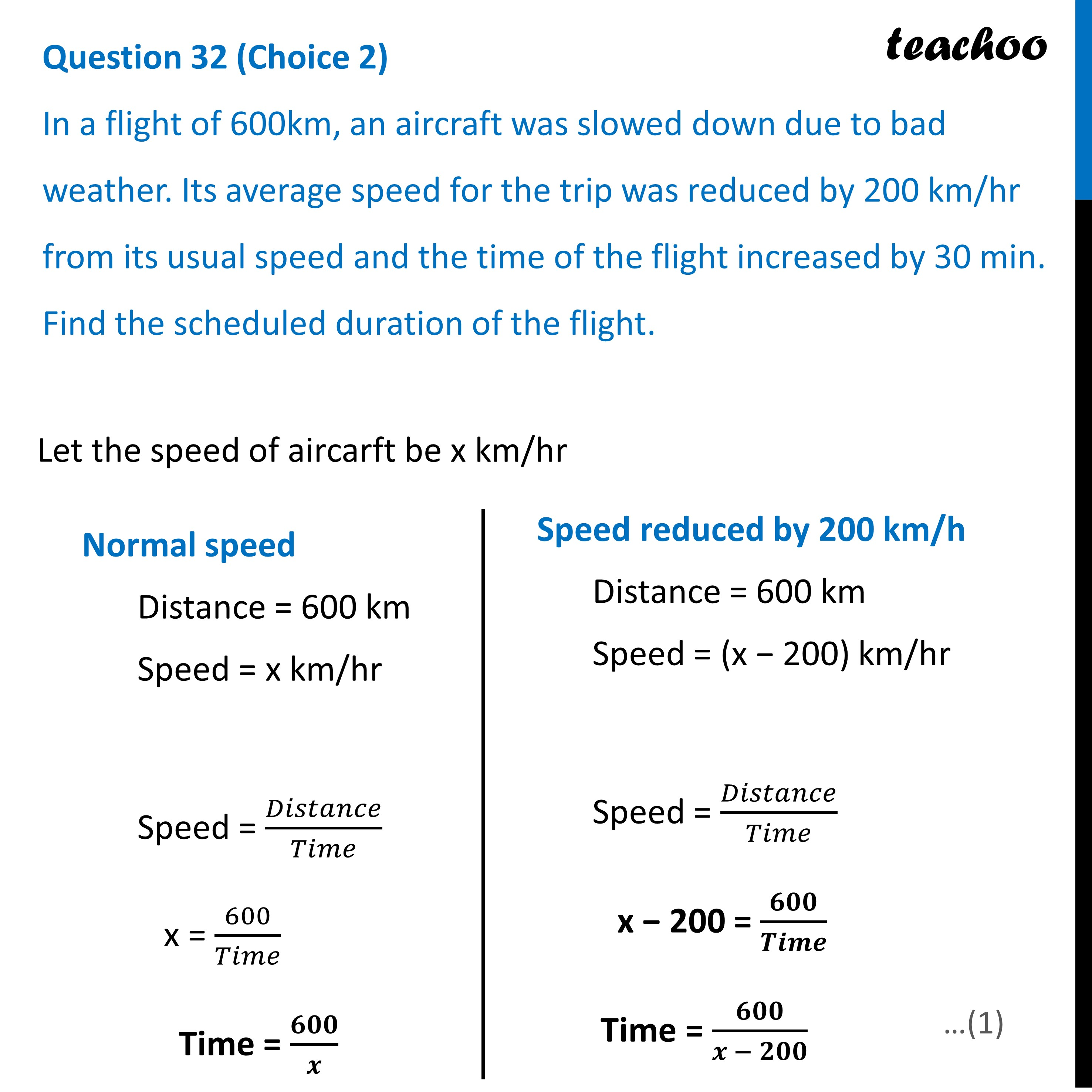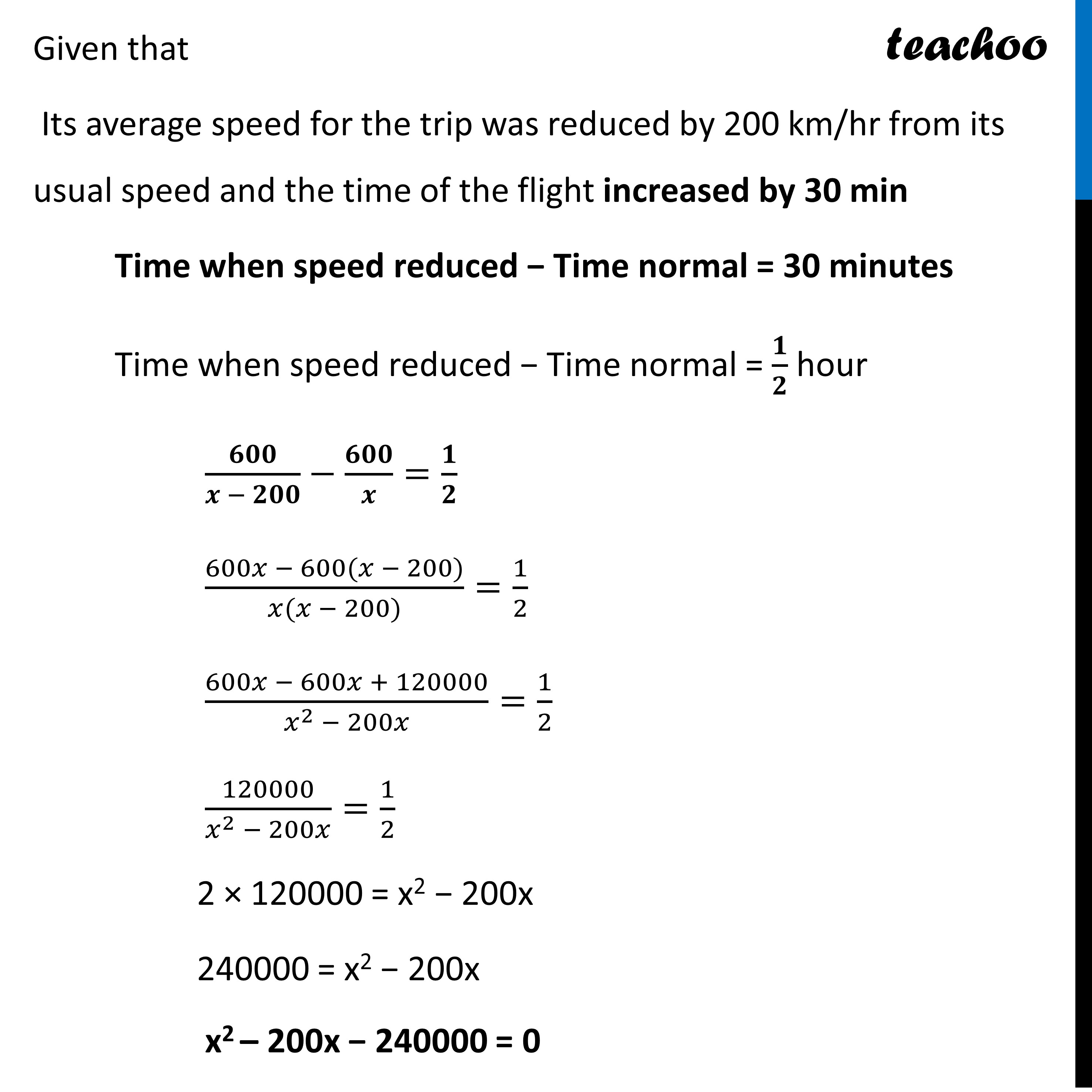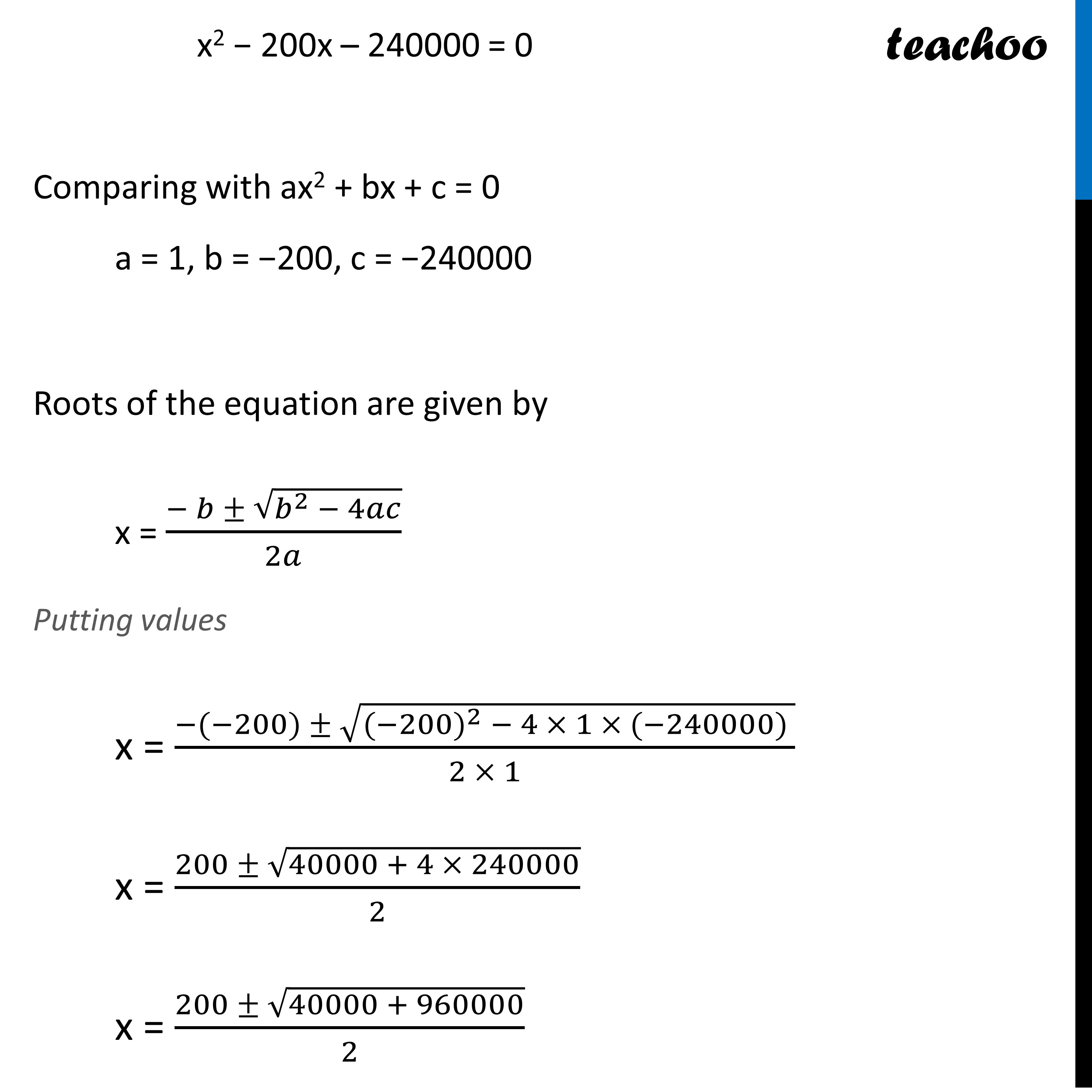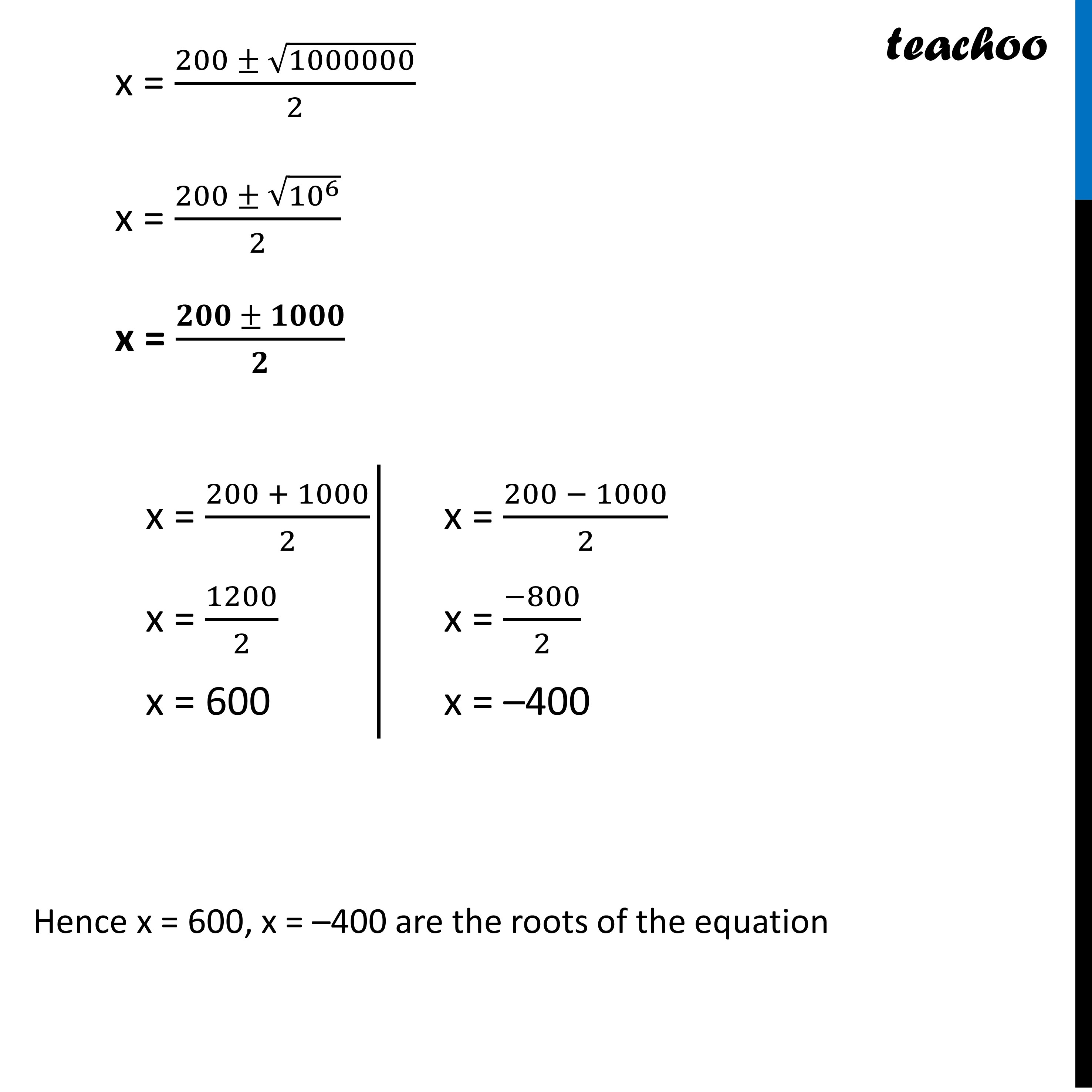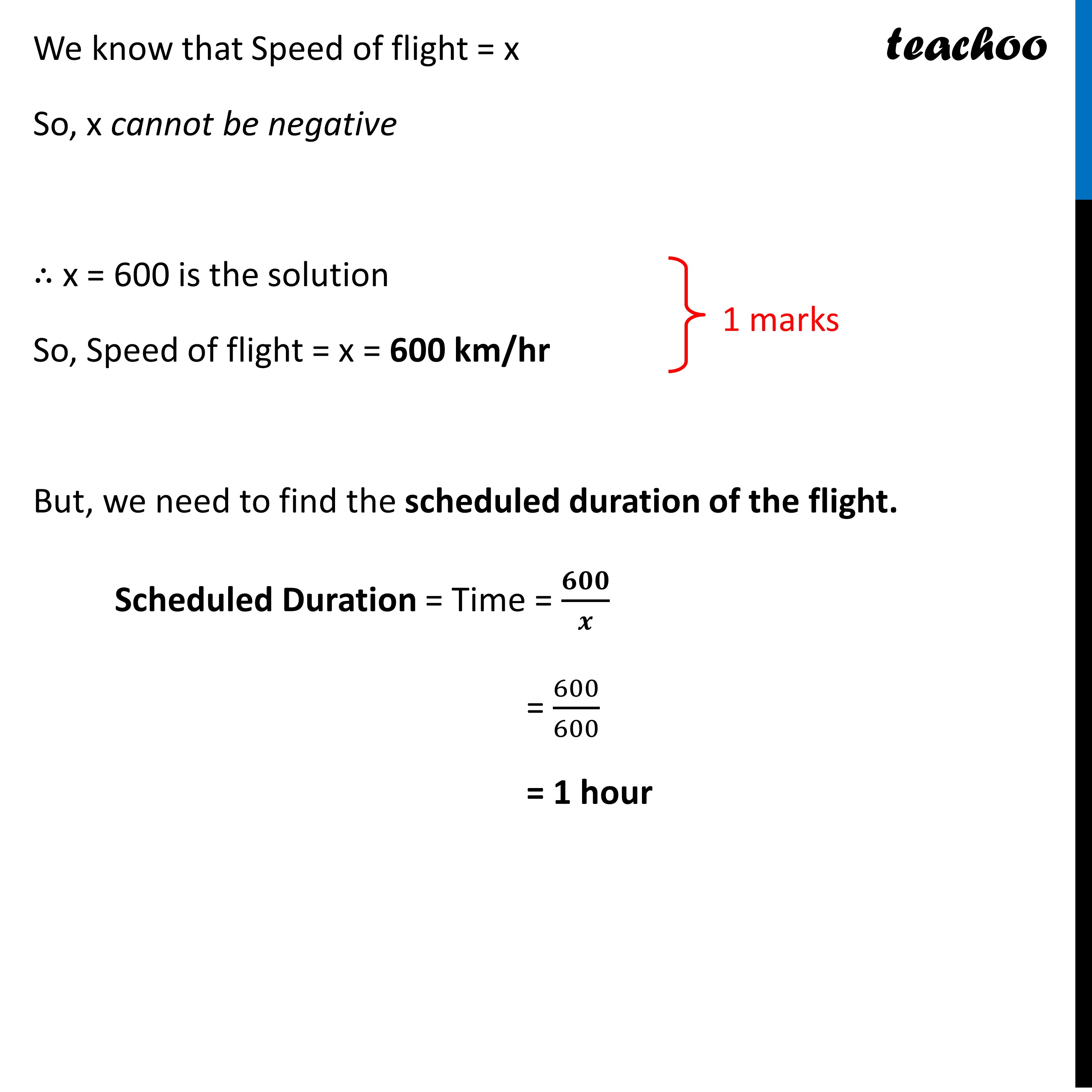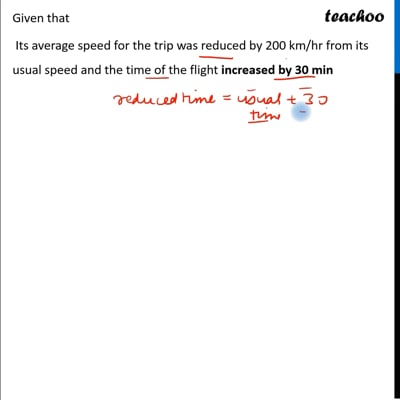This video is only available for Teachoo black users

Learn in your speed, with individual attention - Teachoo Maths 1-on-1 Class

### Transcript

Question 32 (Choice 2) In a flight of 600km, an aircraft was slowed down due to bad weather. Its average speed for the trip was reduced by 200 km/hr from its usual speed and the time of the flight increased by 30 min. Find the scheduled duration of the flight.Let the speed of aircarft be x km/hr Normal speed Distance = 600 km Speed = x km/hr Speed = 𝐷𝑖𝑠𝑡𝑎𝑛𝑐𝑒/𝑇𝑖𝑚𝑒 x = 600/𝑇𝑖𝑚𝑒 Time = 𝟔𝟎𝟎/𝒙 Speed reduced by 200 km/h Distance = 600 km Speed = (x − 200) km/hr Speed = 𝐷𝑖𝑠𝑡𝑎𝑛𝑐𝑒/𝑇𝑖𝑚𝑒 x − 200 = 𝟔𝟎𝟎/𝑻𝒊𝒎𝒆 Time = 𝟔𝟎𝟎/(𝒙 − 𝟐𝟎𝟎) Given that Its average speed for the trip was reduced by 200 km/hr from its usual speed and the time of the flight increased by 30 min Time when speed reduced − Time normal = 30 minutes Time when speed reduced − Time normal = 𝟏/𝟐 hour 𝟔𝟎𝟎/(𝒙 − 𝟐𝟎𝟎)−𝟔𝟎𝟎/𝒙=𝟏/𝟐 (600𝑥 − 600(𝑥 − 200))/(𝑥(𝑥 − 200))=1/2 (600𝑥 − 600𝑥 + 120000)/(𝑥^2 − 200𝑥)=1/2 120000/(𝑥^2 − 200𝑥)=1/2 2 × 120000 = x2 − 200x 240000 = x2 − 200x x2 – 200x − 240000 = 0 x2 − 200x – 240000 = 0 Comparing with ax2 + bx + c = 0 a = 1, b = −200, c = −240000 Roots of the equation are given by x = (− 𝑏 ± √(𝑏^2 − 4𝑎𝑐))/2𝑎 Putting values x = (−(−200) ± √(〖(−200)〗^2 − 4 × 1 × (−240000) ))/(2 × 1) x = (200 ± √(40000 + 4 × 240000))/2 x = (200 ± √(40000 + 960000))/2 x = (200 ± √1000000)/2 x = (200 ± √(〖10〗^6 ))/2 x = (𝟐𝟎𝟎 ± 𝟏𝟎𝟎𝟎)/𝟐 Hence x = 600, x = –400 are the roots of the equation x = (200 + 1000)/2 x = 1200/2 x = 600 x = (200 − 1000)/2 x = (−800)/2 x = –400We know that Speed of flight = x So, x cannot be negative ∴ x = 600 is the solution So, Speed of flight = x = 600 km/hr But, we need to find the scheduled duration of the flight. Scheduled Duration = Time = 𝟔𝟎𝟎/𝒙 = 600/600 = 1 hour